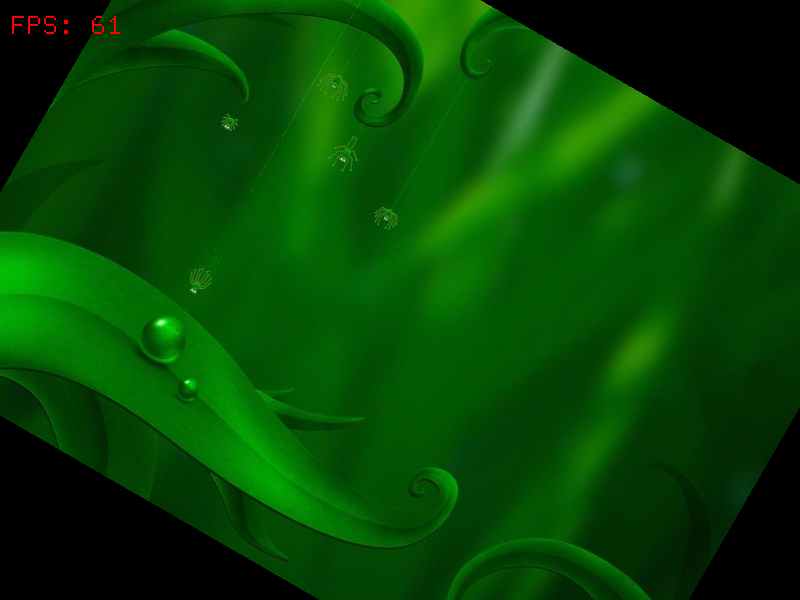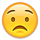# Why smoothing doesn't work?

edited September 2013
When i playing with 01_Object tutorial, i have turn on very smoothing flag display object graphics, but when doing rotate it doesn't work, any idea?

• edited September 2013
Can you please post a screenshot of what your are getting ?
• edited September 2013
If you append this to the end of 01_Object.ini, you should be able to compare them side by side (and press space to take a screenshot):
``````[MainInput]
KEY_SPACE = Screenshot

[Screenshot]
Extension = png

[Object]
Graphic   =
ChildList = O1 # O2 # T1 # T2
TrackList = T

[T]
0         = > Input.IsActive Screenshot # EvalIf < "Screenshot.Capture"
Loop      = true

[O1]
Graphic         = Graphic
Smoothing       = true
Position        = (-200, 0, 0)
AngularVelocity = 90
Scale           = 1.5

[[email protected]]
Smoothing       = false
Position        = (200, 0, 0)

[T1]
Graphic         = @
Pivot           = center top
Text            = @
String          = Smoothing = true
Position        = (-200, 200, 0)

[[email protected]]
String          = Smoothing = false
Position        = (200, 200, 0)
``````
• edited September 2013
; orx - Tutorial config file
; Should be used with orx v.1.4+

[Display]
;ScreenWidth = 1366
;ScreenHeight = 768
ScreenWidth = 800
ScreenHeight = 600
Title = SharpDawn
;FullScreen =true
;Smoothing =true
;DepthBuffer =true

[Input]
SetList = MainInput

[MainInput]
KEY_ESCAPE = Quit
KEY_SPACE = Screenshot

[Render]
;nFrequency = <float>
ShowFPS = true
;ShowProfiler = true
;onsoleColor = <vector>

[Viewport]
Camera = Camera
;RelativePosition = bottom right
;Position = (100, 100, 0); NB: Position is in pixels and will be ignored if RelativePosition is specified
;RelativeSize = (0.5, 0.5, 0.0)
;Size = (200, 150, 0) NB: Size is in pixels and will be ignored if RelativeSize is specified
;BackgroundColor = (255, 180, 0)

[Camera]
; We use the same size for the camera than our display on screen so as to obtain a 1:1 ratio
FrustumWidth = @Display.ScreenWidth
FrustumHeight = @Display.ScreenHeight
;FrustumWidth = 2000
;FrustumHeight = 300
FrustumFar = 1.0
FrustumNear = 0.0
Position = (0.0, 0.0, -1.0)
;Zoom = 0.9
;Rotation = 35

[Object]
Graphic = Graphic
;AutoScroll = x
;Color = (255, 0, 200)
;Alpha = 0.4
;Flip = both
;Position = (100.0, 200.0, 0.0)
Rotation = 30
;Scale = (3.0, 1.0, 1.0) NB: z is ignored for 2D objects
;Smoothing = true
;AngularVelocity = 1800
Speed = (0, 0, 0)
TrackList = T

[Text]
String = Why should we use a texture when we can use a text?!

[Graphic]
Texture = ../data/object/bg.jpg
;Text = Text; <= If you want to see the text, you need to comment out the texture
Pivot = center
;TextureCorner = (50, 20, 0) NB: z is ignored
;TextureSize = (50, 80, 0) NB: z is ignored
;Repeat = (3.0, 2.0, 0.0)
;Flip = both
;Color = (0, 0, 255)
;Alpha = 0.6
Smoothing = true

[Screenshot]
Extension = png

[T]
0 = > Input.IsActive Screenshot # EvalIf < "Screenshot.Capture"
Loop = true• edited September 2013
This is what i play with the tutorial, when rotate the edge of the picture seems no smooth as expectwith wrong config?
• edited September 2013
That's very known issue while rotating square texture with no alpha border, try to insert a border of alpha pixel or transparent pixel in your image using photoshop or gimp. This would smoothen the edge a lot. So if you image size is 16x16, make it 17x17 with transparent pixel around the border.

edit : 17x17 wont work for 16x16 image, you need 18x18, 1 pixel on each side of your image
• edited September 2013
Ah I see from where the confusion is coming.The smoothing attribute corresponds to texture filtering (ie. hardware interpolation done inside the texture surface while sampling), not to anti-aliasing.

You can use Jim's trick in order to get less rough edges (ie. having filtering on those extra rows of pixels) or you can integrate any anti-aliasing technique that would work for you, such as FXAA (which is a post-processing shader).